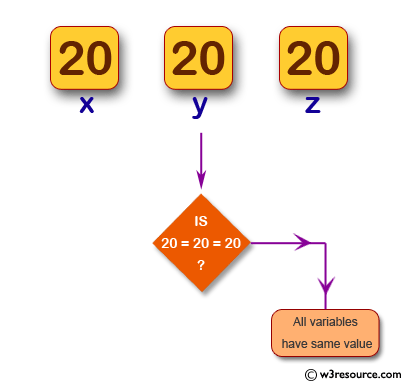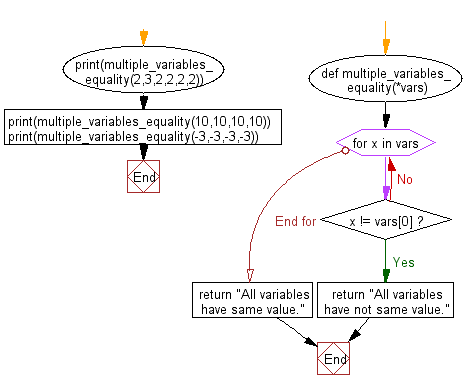﻿ Python: Check whether multiple variables have the same value - w3resource# Python: Check whether multiple variables have the same value

## Python Basic: Exercise-124 with Solution

Write a Python program to check whether multiple variables have the same value.

Pictorial Presentation:Sample Solution-1:

Python Code:

``````x = 20
y = 20
z = 20
if x == y == z == 20:
print("All variables have same value!")
```
```

Sample Output:

```All variables have same value!
```

## Visualize Python code execution:

The following tool visualize what the computer is doing step-by-step as it executes the said program:

Sample Solution-2:

Python Code:

``````def multiple_variables_equality(*vars):
for x in vars:
if x != vars:
return "All variables have not same value."
return "All variables have same value."
print(multiple_variables_equality(2,3,2,2,2,2))
print(multiple_variables_equality(10,10,10,10))
print(multiple_variables_equality(-3,-3,-3,-3))
```
```

Sample Output:

```All variables have not same value.
All variables have same value.
All variables have same value.
```

Flowchart:## Visualize Python code execution:

The following tool visualize what the computer is doing step-by-step as it executes the said program:

Python Code Editor:

Have another way to solve this solution? Contribute your code (and comments) through Disqus.

What is the difficulty level of this exercise?

Test your Python skills with w3resource's quiz

﻿

## Python: Tips of the Day

Unpack variables from iterable:

```# One can unpack all iterables (tuples, list etc)
>>> a, b, c = 1, 2, 3
>>> a, b, c
(1, 2, 3)

>>> a, b, c = [1, 2, 3]
>>> a, b, c
(1, 2, 3)
```